# Current, Resistance, Voltage, and Power

Current
Current is a measure of the flow of electric charge through a material. A material that can carry a flow of charge is called a conductor. Current is defined as the amount of charge that flows through a conductor in a certain amount of time. The unit of current is the Ampere (A), which is equal to one Coulomb per second (Coulomb is the unit of charge),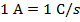The symbol I is used to represent current (though J is often used in engineering sources). The current I through a conductor depends on its area A, the concentration n of charge carriers, the magnitude of the charge q of each carrier, and the magnitude of their average (or "drift") velocity vd,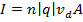Current density is the amount of current flowing through a conductor divided by its area,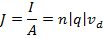The direction of the flow of current is defined in terms of a flow of positive charges (even if the actual charge carriers are negative). The unit of current density is Amperes per meter squared (A/m2).

Resistivity
Some conductors carry charge more easily than others. The resistivity of a material describes how easily charge can flow. Good conductors have a small resistivity, and good insulators have a large resistivity. The resistivity ρ (the Greek letter "rho") is equal to magnitude of the electric field in the material divided by the current density,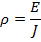The unit for the magnitude of an electric field is a Volt per meter (V/m), and the unit of current density is an Ampere per meter squared (A/m2), and so the unit for resistivity is a Volt-meter per Ampere,Many conductors follow Ohm's Law. Materials that follow Ohm's law have a constant resistivity no matter what the values of the electric field E and current density J are. The formulas related to circuits are true for "Ohmic" materials, and "non-Ohmic" materials are not discussed in this course.

The resistivity of an Ohmic conductor depends on the temperature of the material. The temperature-dependent resistivity ρ(T) can be found using the formula,This formula requires ρ0, the resistivity at a reference temperature T0. The temperature coefficient of resistivity α is different for every material. For temperatures in degrees Celsius (℃), the temperature coefficient of resistivity has units 1/℃ = (℃)(-1)

Resistance
Resistivity is a property of a type of material, but resistance is a property of a certain piece of that material. The resistance of a piece of conductor depends on its length L, area A, and resistivity ρ,The unit of resistance is the Ohm, which is represented with the Greek letter Ω ("omega"). One Ohm is equal to one Volt per Ampere,Resistance depends on temperature in the same way as resistivity,This formula requires R0, the resistance at a reference temperature T0. The temperature coefficient α is different for every material, as described in the Resistance section.

A resistor is a device that is used in electric circuits, and has a certain fixed resistance. Resistors are made by choosing a piece of material with a certain resistivity, length, and area, and wrapping it in an insulator with wires leading out of each end. In circuit diagrams, it is represented with the symbol,Voltage
Voltage is a difference in electric potential between two points. If an electric field is uniform through a conductor, the potential difference is,Using equations in the Current, Resistivity, and Resistance sections, another equation for the potential difference can be found,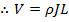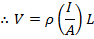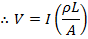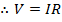The equation V = IR means that the potential difference, or voltage, across a resistor can be found by multiplying its resistance by the current flowing through it. The unit of potential difference is the Volt (V), which is equal to a Joule per Coulomb (J/C).

A voltage source is a device used in electric circuits that has a fixed potential difference between its ends. A voltage source can be a battery, or another source of direct current with a fixed potential difference. In circuit diagrams, it is represented with the symbol,If the ends of a voltage source are connected through a circuit with any number of resistors or other components, a complete circuit is formed, and current can flow from one terminal to the other. If current is flowing, it will be the same on both terminals of the voltage source.

A voltage source that is part of a complete circuit can produce an electromotive force, which is represented with the symbol ε ("script e"). The unit of electromotive force is the Volt (V), which is equal to a Joule per Coulomb (J/C). For an ideal source, the electromotive force is equal to the voltage difference,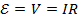Real sources like batteries are not ideal, and so there is some amount of internal resistance. If the internal resistance of a battery is r, then the voltage difference across the battery is,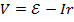This is also called the terminal voltage of the battery. If a complete circuit is made using a resistor with a resistance R, the current flowing through the circuit can be found by using the equation V = IR,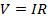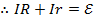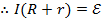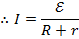The current is equal to the electromotive force of the source divided by the total circuit resistance.

Power
Power (P) is a measure of the rate at which energy is delivered or used by a circuit element. Voltage sources deliver power, while resistors use power (by dissipating it as heat). Power is equal to the voltage across a circuit element multiplied by the current flowing through it,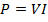The unit for power is the Watt (W), which is equal to a Joule per second,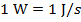This relation can be found from the formula for power,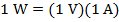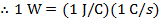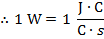The power used or dissipated by a resistor can be found using the formula V = IR. This formula can be used to substitute for the voltage or for the current in the power formula,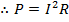,

and,The power output of a battery that has internal resistance can be found using the formula V = ε-Ir and the formula for power,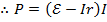Related Links: Physics Quizzes AP Physics Notes Displacement, Velocity, and Acceleration